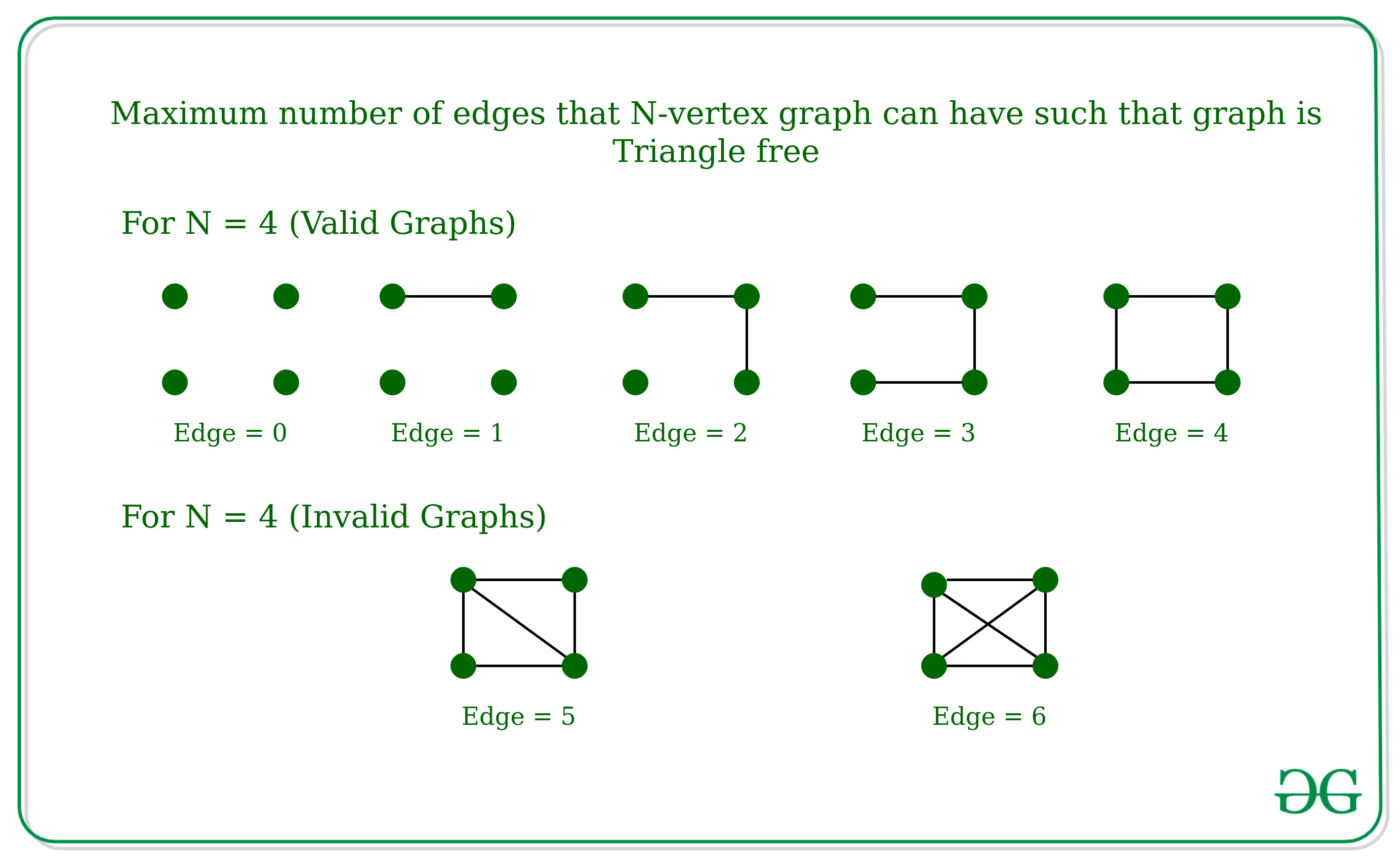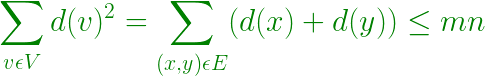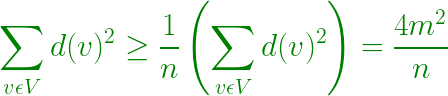# Maximum number of edges that N-vertex graph can have such that graph is Triangle free | Mantel’s Theorem

Given a number N which is the number of nodes in a graph, the task is to find the maximum number of edges that N-vertex graph can have such that graph is triangle-free (which means there should not be any three edges A, B, C in the graph such that A is connected to B, B is connected to C and C is connected to A). The graph cannot contain a self-loop or multi edges.

Examples:

Input: N = 4
Output:
Explanation:Input: N = 3
Output:
Explanation:
If there are three edges in 3-vertex graph then it will have a triangle.

Approach: This Problem can be solved using Mantel’s Theorem which states that the maximum number of edges in a graph without containing any triangle is floor(n2/4). In other words, one must delete nearly half of the edges to obtain a triangle-free graph.

How Mantel’s Theorem Works ?
For any Graph, such that the graph is Triangle free then for any vertex Z can only be connected to any of one vertex from x and y, i.e. For any edge connected between x and y, d(x) + d(y) ? N, where d(x) and d(y) is the degree of the vertex x and y.

• Then, the Degree of all vertex –• By Cauchy-Schwarz inequality –• Therefore, 4m2 / n ? mn, which implies m ? n2 / 4

Below is the implementation of above approach:

## C++

 `// C++ implementation to find the maximum` `// number of edges for triangle free graph`   `#include ` `using` `namespace` `std;`   `// Function to find the maximum number of` `// edges in a N-vertex graph.` `int` `solve(``int` `n)` `{` `    ``// According to the Mantel's theorem` `    ``// the maximum number of edges will be` `    ``// floor of [(n^2)/4]` `    ``int` `ans = (n * n / 4);`   `    ``return` `ans;` `}`   `// Driver Function` `int` `main()` `{` `    ``int` `n = 10;` `    ``cout << solve(n) << endl;` `    ``return` `0;` `}`

## Java

 `// Java implementation to find the maximum ` `// number of edges for triangle free graph ` `class` `GFG ` `{ `   `    ``// Function to find the maximum number of ` `    ``// edges in a N-vertex graph. ` `    ``public` `static` `int` `solve(``int` `n) ` `    ``{` `        `  `        ``// According to the Mantel's theorem ` `        ``// the maximum number of edges will be ` `        ``// floor of [(n^2)/4] ` `        ``int` `ans = (n * n / ``4``); `   `        ``return` `ans; ` `    ``} `   `    ``// Driver code` `    ``public` `static` `void` `main(String args[])` `    ``{ ` `        ``int` `n = ``10``; ` `        ``System.out.println(solve(n)); ` `    ``} ` `}`   `// This code is contributed by divyamohan123`

## C#

 `// C# implementation to find the maximum ` `// number of edges for triangle free graph ` `using` `System;`   `class` `GFG ` `{ `   `    ``// Function to find the maximum number of ` `    ``// edges in a N-vertex graph. ` `    ``public` `static` `int` `solve(``int` `n) ` `    ``{` `        `  `        ``// According to the Mantel's theorem ` `        ``// the maximum number of edges will be ` `        ``// floor of [(n^2)/4] ` `        ``int` `ans = (n * n / 4); `   `        ``return` `ans; ` `    ``} `   `    ``// Driver code` `    ``public` `static` `void` `Main()` `    ``{ ` `        ``int` `n = 10; ` `        ``Console.WriteLine(solve(n)); ` `    ``} ` `}`   `// This code is contributed by AnkitRai01`

## Python3

 `# Python3 implementation to find the maximum` `# number of edges for triangle free graph`   `# Function to find the maximum number of` `# edges in a N-vertex graph.` `def` `solve(n):` `    `  `    ``# According to the Mantel's theorem` `    ``# the maximum number of edges will be` `    ``# floor of [(n^2)/4]` `    ``ans ``=` `(n ``*` `n ``/``/` `4``)`   `    ``return` `ans`   `# Driver Function` `if` `__name__ ``=``=` `'__main__'``:` `    ``n ``=` `10` `    ``print``(solve(n))`   `# This code is contributed by mohit kumar 29`

## Javascript

 ``

Output:

`25`

Time Complexity: O(1)